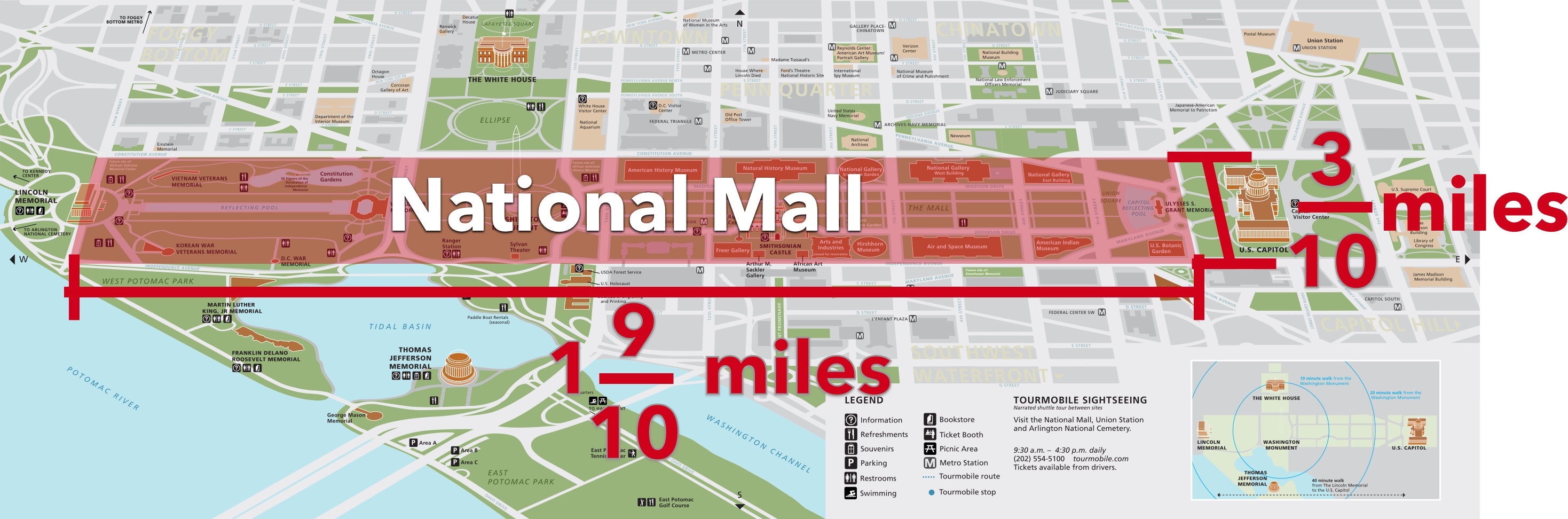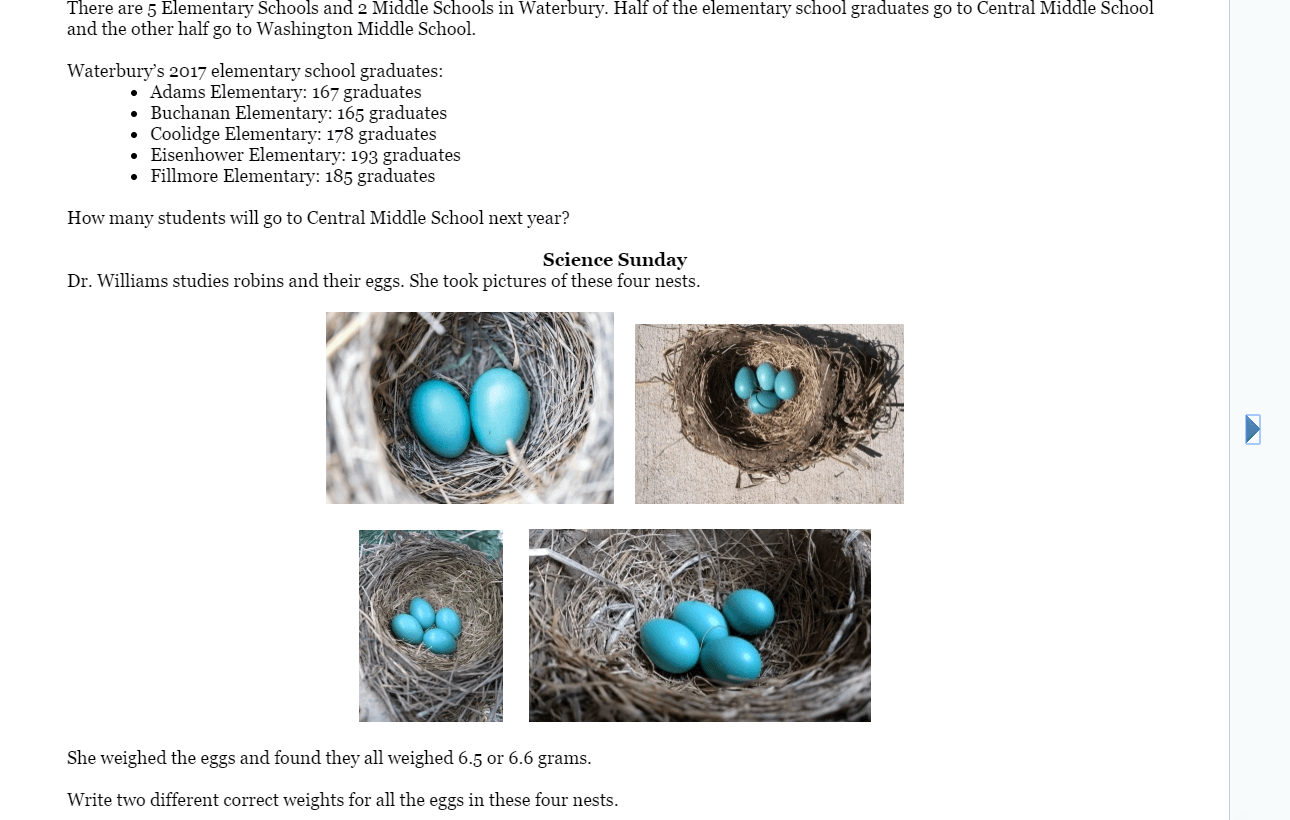# Summer Mathness 6

Welcome to the Summer Mathness 6 page!

You can always buy the book at Amazon.You can enter comments below that link to pictures, YouTube videos and descriptions of your answer and how you found it. Please also support and give good feedback to others working on these problems.

Please note that we will not post our feedback and solutions before the day listed in your book.

Thanks and happy summer!

## 9 thoughts on “Summer Mathness 6”

1. Sat. June 17: I added all the students then divided: 888 / 2 = 444

2. 6/16. Friday

The fractions add up to less than 1, so the recipe is between 5 and 6 cups. I would use the blue bowl.

4 times the recipe is

6+5+4+4+4= 23 cups

3. Thursday. 6/16.

=\$5.74

4. Wednesday, June 14:

In this picture, we added the quarter miles then multiplied by 177.

You could also multiply 0.25 miles by 177 x 2 = 354 trips.

Both answers are equal to 88.5 miles.

1. I also added 1/4 + 1/4=1/2. Multiplying by 1/2 can be calculated by dividing by 2

177 / 2 = 88 1/2

5. Tuesday, June 13:

You could see one calculation in this picture.

Or, convert the numbers into decimals, so that 1.9 miles x 0.3 miles = 0.57 miles.

1. I broke it into a rectangle that was 1 mile by 0.3 miles and .9 miles by 0.3 miles. So, the total area is 0.3 square miles and 0.27 square miles.

6. Monday, June 12:

There are many ways to solve this one. We showed how to calculate the total costs and then subtract. OR, you could just multiply the difference in costs by the number purchased.

1. I got the same answers using my calculator to multiply

\$37.62

\$3.80